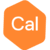# VOXI, Why the "predicted data" not equal to "Gz_FWD" when i use a same model??Posts: 3edited September 2017
Hi

I'm trying to extract the inverted model from VOXI and then input it for FW calculation in "starting model". The problem is that the predicted data from VOXI (the Fw calculation data) is unequal to the Gz_FWD (Fw calculation). These two gravity data (predicted data and Gz_FWD) also showed the difference value average of 4 mGal. Is it seem to the DC-Shift in GM-SYS module??

• Hi,

Were your mesh and padding cells exactly the same when you ran the separate forward calculation?

To be sure, use the same VOXI project with the same mesh as the inversion. Be sure to export the inversion result WITH padding cells, and use that in the forward calculation.
Customer Success Manager - Geophysical Modelling•Posts: 3Thank you, TaronishPithawala

To make sure that my understanding is correct, these gravity data (Gz_FWD and predicted data) normally should be same value?.
• Hi @NitiMankhemthong ,

Assuming all else is the same, then yes the two values (Gz_FWD and Predicted Data) should be equal.
Customer Success Manager - Geophysical ModellingThis discussion has been closed.